# Identification of Photovoltaic Panel MPPT Using Neuro-Fuzzy Model

Identification of Photovoltaic Panel MPPT Using Neuro-Fuzzy Model

Aouiche AbdelazizAouiche El Moundher Aouiche Chaima Djellab Hanane

Department of Electrical Engineering, LABGET Laboratory, Faculty of Sciences and Technology, Echahid Cheikh Larbi Tebessi University, Tebessa 12000, Algeria

School of Electrical and Electronic Engineering, Hebei University of Technology, Tianjin 300130, China

Department of Electrical Engineering, (LAMIS) Laboratory of Mathematics, Informatics and Systems, Faculty of Sciences and Technology, Echahid Cheikh Larbi Tebessi University, Tebessa 12000, Algeria

Corresponding Author Email:
abdelaziz.aouiche@univ-tebessa.dz
Page:
273-279
|
DOI:
https://doi.org/10.18280/ejee.245-606
16 November 2022
|
Accepted:
16 December 2022
|
Published:
31 December 2022
| Citation

OPEN ACCESS

Abstract:

A photovoltaic (PV) panel produces energy that is influenced by external factors including temperature, irradiation, and the fluctuations in the load related to it. The PV system should perform at maximum power point (MPP) in order to adjust towards the rapidly increasing interest in energy. Because of the changing climatic conditions, it becomes has a limited efficiency. In order to maximize the PV system's efficiency, a maximum power point technique is necessary. In the present paper a maximum power point (MPP) of photovoltaic (PV) panel is designed and simulated to optimize system performance, accurate synthesis model based on the hybrid neural fuzzy systems is proposed to directly obtain the MPP. So, photovoltaic panel (PV) is analyzed with the mathematical model to obtain the training data. Three cases were used to test the identification of the structure proposed. The results show neuro-fuzzy (Sugeno Model) used were efficient in modeling the MPP of our PV panel. The Mean square error (MSE) is used as the fitness function to guarantee that the MSE is small, the algorithm synthesis model is validated by the MPP PV Panel analysis, simulation, and measurements. Neuro-fuzzy models is presented throughout this paper to demonstrate the effectiveness of the method of training suggested.

Keywords:

fuzzy inference systems, maximum power point tracking, modeling, photovoltaic panel

1. Introduction

Although a solar panel's ability to absorb solar energy is unaffected by temperature, it does influence how much power is generated. Less power generated from the same amount of sunshine when the solar panels get hotter. The voltage differential  that you may theoretically obtain from the solar panel is the result of electrons being at rest (low energy) and being agitated by the sun (high energy). However, temperature also agitates electrons (by warming something, we provide it energy), increasing the energy of the electrons at rest. ("warmer" electrons offer more energy at rest compared to their "cold" equivalents). So, the geographic distribution of photovoltaic energy potential is taken into account, as well as the influence of irradiance and temperature on PV panel performance [1-3].

However, The Maximum Power Point (MPP) is the point at which the panel should be operating optimally, so the optimum system performance requires real-time control of the (MPP).

Several MPPT methods have been suggested and used in practice. Included among them are the incremental conductance (Inc-Con), the perturb and observe (P&O), fractional short-circuit current and fuzzy set system (FS). Some updated methods which attempt to reduce the amount of hardware required or to increase performance have also been presented forth [4-6]. These traditional MPPT techniques can offer better performance measurement when the PV Panel's circumstances are stable. but when the atmospheric conditions change rapidly, the traditional techniques may perform substantially worse. Therefore, many researchers have tried to apply intelligent MPP approaches that are effective for conditions that change quickly. They have become an interesting alternative to the conventional or traditional approaches to harvest maximum power from a PV panel [7, 8].

The suggested intelligent solution in this study is more cheap, adaptable, and very efficient; The Adaptive Network-based Fuzzy Inference System (ANFIS) with its architecture and algorithm, to reach the MPP even when environmental factors are the worst .

The ANFIS is a kind of artificial neural network designed on the Takagi-Sugeno fuzzy inference system (FIS). It mixes the advantages of both FISs and artificial neural networks (ANNs) into a unified architecture. Excellent explanation tools in the form of semantic representations fuzzy rules, rapid and precise learning, the capacity to take into account data as well as the problem's existing expert knowledge, neuro-fuzzy systems have become more popular in recent years because to their advantages for generalization and other aspects . So, in this work ANFIS modeling technique is introduced to determine PV Panel MPPT .

The suggested MPPT technique is verified, validated, and simulated using the Matlab software platform while temperature and irradiance are dynamically changed. In comparison to existing conventional-based MPPT methods, the suggested ANFIS's MPPT performance is superior. The proposed method is tested with three different cases; the first one when the irradiation varies and the temperature stays constant, the second case when the temperature varies and the irradiation is constant and the last case is the difficult one when the temperature and the irradiation change together. The simulation results show that the ANFIS can replace the real model of PV panel in terms of delivering better efficiencies and rise time, with and without the appearance uncertainties in the model and the tracking error between the model and the system was decreased to a minute level.

This paper is divides in four sections, the second section describes the process that modelling MPPT, the design of ANFIS is explained shortly in section 3, and then the implementation of this technique to the tracking of MPP is showed and simulated. At the end of this paper, we will show how our selected method can achieve very robust and satisfactory performance.

2. Background

2.1 Solar cell equivalent model

The electronic component that transforms solar energy into electricity is considered as a cell [11, 12]. A Photovoltaic Panel (PV) module is an array of cells that have been connected in series, there are parallel and series connections between the modules. The majority of mathematical models that have been created are based on the single-diode model's current-voltage relationship as shown in the following Figure 1, which makes the assumption PV cell's characteristics can be described by a single lumped diode mechanism .

## 1.png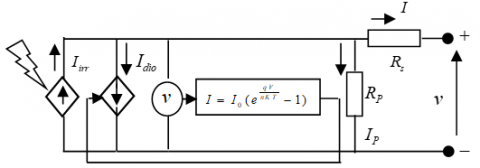Figure 1. Equivalent solar cell circuit

By the current law of Kirchoff:

$I=I_{i r r}-I_{d i o}-I_p$     (1)

$I_{i r r}$: is the irradiance current or the photo-current, where, for a given cell temperature, is produced after the cell has been exposed to direct sunlight.

The solar cell's non-linear properties are produced by the current passing through the anti-parallel diode $I_{\text {dio }}$.

where,

$I_{d i o}=I_0\left\{\exp \left(\frac{q\left(v+I R_s\right)}{n K T}\right)-1\right\}$     (2)

The shunt current $I_P$ given to the branch of the shunt resistor $R_P$ is given as follow:

$I_P=\left(\frac{v+I R_s}{R_P}\right)$     （3）

Substitution the important expressions of $I_{\text {dio }}$ and $I_P$,

$I=I_{i r r}-I_0\left\{\exp \left(\frac{q\left(v+I R_s\right)}{n K T}\right)-1\right\}-\left(\frac{v+I R_s}{R_P}\right)$    (4)

where,

q =1.602 × 10−19 C is the electronic charge,

K =1.3806503 × 10−23 J/K is the Boltzmann constant,

n: is the ideal constant of the diode,

T: is the cell's temperature,

$I_0$: is the diode saturation current,

$R_P$ and $R_s$ represent the shunt and series resistance, respectively .

2.2 Photovoltaic module current-voltage relationship

A PV module typically consists of several solar cells connected in series. $N_S$ denotes the quantity of solar cells arranged in series for a single module.

As an illustration: $N_S=36$ for BP Solar's BP 365 Module, $N_S=$ 72 for ET-Solar's ET Black Module ET-M572190BB etc. When $N_S$ solar cells constructed into a module by connecting them in series, the module's output voltage $V_M$ and output current $I_M$ have the following relationships:

$I_M=I_{i r r}-I_0\left\{\exp \left(\frac{q\left(V_M+I_M N_S R_s\right)}{N_S n K T}\right)-1\right\}$$-\left(\frac{V_M+I_M N_S R_s}{N_S R_P}\right)$      (5)

This equation can be expanded to any number of cells in series ($N_S$), hence it is not constrained to only one module. If each module has NC cells in series and $N_M$ modules are connected in series, then :

$N_S=N_M \times N_C$

2.3 Basic model parameters

It is important to talk about the primary model characteristics and how they vary with operational situations.

Irradiance and temperature both influence the photocurrent (6):

$I_{p h}=I_{i r r, r e f}\left(\frac{G}{G_{r e f}}\right)\left[1+\alpha_T^{\prime}\left(T-T_{r e f}\right)\right]$      (6)

$G$: Irradiance $\mathrm{W} / \mathrm{m}^2$

$G_{\mathrm{ref}}$: Irradiance at 1000 W/m2,

where $I_{\text {irr }, r e f}$  is the photo current at SRC,

$\alpha_T^{\prime}$ is the short-circuit current's relative temperature coefficient, which shows how quickly the short-circuit current changes as a function of temperature. The absolute temperature coefficient of the short-circuit current is infrequently provided by manufacturers $\alpha_T$ for a particular panel [15-17].

The relationship between $\alpha_T^{\prime}$ and $\alpha_T$ is

$\alpha_T=\alpha_T^{\prime} \times I_{i r r, r e f}$

$I_{\text {irr }, \text { ref }}$: is the second unknown parameter in the model.

$I_0$: is mostly influenced by the cell's temperature:

$I_0=I_{0, \text { ref }}\left[\frac{T}{T_{\text {ref }}}\right]^3 \exp \left[\frac{E_{g, \text { ref }}}{k T_{\text {ref }}}-\frac{E_g}{k T}\right]$    （7）

T: Cell temperature in its actual state (K)

$T_{\text {ref }}$: Cell temperature at $25^{\circ}$

$I_{0, r e f}$: The third undefined model’s parameter is the saturating diode current during cell temperature at SRC.

$E_g$: the bandgap energy [eV] defined as:

$E_g=1.16-7.02 \times 10^{-4}\left(\frac{T^2}{T-1108}\right)$      (8)

The power-voltage derivative is equal to zero at the point of maximum power in the SRC

$\frac{\partial p}{\partial v} \mid p=p_{\max , S R C=0}\left(P=V_A \times I_A\right)$     (9)

Normative test conditions or the nominal condition ($\operatorname{STC}_s$) of solar irradiation and temperature are always used to produce this information [18, 19].

Some manufacturers provide I-V curves for several irradiation and temperature conditions. These curves make easier the adjustment and the validation of the desired mathematical I-V equation. Basically, the Table 1 presents all the information one can get from datasheet of PV Panel) [20, 21].

Table 1. Electrical parameters of the BP SX 150S PV array at 25℃,1000W/m2

 Electrical characteristics Value Maximum Power ($P_{m p p}$) 150 W Voltage at $P_{m p p}$  ($V_{m p p}$) 34.5 V Current at $P_{m p p}$  ($I_{m p p}$) 4.35 A Short-circuit current ($I_{s c}$) 4.75 A Open-circuit voltage ($V_{o c}$) 43.5 V Temperature coefficient of $I_{s c}$ (0.065$\pm$0.015)%/℃ Temperature coefficient of $V_{o c}$ -(160$\pm$20)mV/℃ Number of cells series ($N_S$ ) 72
3. Maximum Power Point Tracking (MPPT)

The most famous conventional MPPT methods are the Perturb and Observe (Hill climbing) method [22, 23], the Incremental Conductance method [24, 25], the Fractional open circuit voltage method [26, 27] and the Fractional short circuit Current method [28, 29]. And the artificial intelligence methods Fuzzy logic based MPPT  and Neural Networks based ones  and hybrid neuro-fuzzy technique is the objective of this paper.

4. Neuro-Fuzzy Network Structure

ANFIS give better solutions to nonlinear problems because it merges fuzzy logic and neural networks approaches. It may fast arrive to the best possible result even if the targets are not defined .

In our study a five-layer neuro-fuzzy network is used to reach MPP, the selected ANFIS architecture is shown in Figure 2 based on the empirical analysis to obtain the final model who performs very well as predicting output data. An optimal ANFIS model consists of 2 input variables irradiance (E) and temperature (T), two membership functions and minimum number of rules, in our study we selected only two rules, the output of our ANFIS model is the maximum power of MPP ($P_{m p p}$). Whereas the circular nodes indicate nodes that are fixed.

## 2.png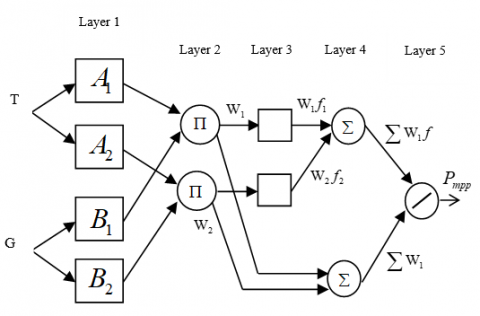Figure 2. ANFIS architecture for a Sugeno system with two rules

Our Sugeno ANFIS model contains the following two rules:

Rule 1: If $x$ is $A_1$ and $y$ is $B_1$ THEN $f_1=p_1 x+q_1 y+r_1$

Rule 2: If $x$ is $A_2$ and $y$ is $B_2$ THEN $f_2=p_2 x+q_2 y+r_2$

There are forward and backward passes possible for the network's training.  Now, we check each layer separately for the forward pass. The input vector is passed forward, propagating layer by layer through the network.  Similar to back-propagation, the error is propagated back through the network during the backward pass .

Layer 1

Each node's output is:

$O_{1, i}=\mu_{A_i}(x) \quad$ for $i=1,2$    (10)

$O_{1, i}=\mu_{B_{i-2}}(y) \quad$ for $i=3,4$    （11）

So, the $O_{1, i}(x)$  is essentially the membership grade for x and y.

The membership functions could be anything but for illustration purposes we will use the bell-shaped function given by:

$\mu_A(x)=\frac{1}{1+\left|\frac{x-c_i}{a_i}\right|^{2 b_i}}$     (12)

where, the premise parameters to be learned are $a_i, b_i$ and $c_i$.

Layer 2

In this layer, each node is fixed. Here, the t-norm is applied to 'AND' the membership functions; as the following product:

$O_{2, i}=w_i=\mu_{A_i}(x) \mu_{B_i}(y), \quad i=1,2$     (13)

Layer 3

This layer has fixed nodes which determines the firing strength ratio according to the rules:

$O_{3, i}=\overline{w_l}=\frac{w_i}{w_1+w_2}$    (14)

Layer 4

The nodes in this layer are adaptive and perform the consequent of the rules:

$O_{4, i}=\overline{w_i} f_i=\overline{w_i}\left(p_i x+q_i y+r_i\right)$     (15)

The parameters in this layer $\left(p_i, q_i, r_i\right)$ are the consequent parameters and must be determined.

Layer 5

Here, the complete output function is performed by a single node :

$O_{5, i}=\sum_i \overline{w_i} f_i=\frac{\sum_i w_i f_i}{\sum_i w_i}$    (16)

We verify that the neuro-fuzzy models after learning are actually able to predict the desired output for values given at the entry which are not used in the learning. We always should compare the true output of the networks using V-I properties of the desired PV (Tab 1) for comparisons.

The training data sets were obtained from PV Panel with about 432 data were used to train the neuro-fuzzy models.

To train the model, some data must be obtained for the input and output variables. As a consequence, the distinct layers of the neuro-fuzzy model acquire their rules. To obtain data, PV model coding in MATLAB is performed.

The rules have been specified after the fuzzy model has been trained, for any $T$ and $E$ inputs, and $P_{m p p}$ output of our ANFIS. Now, likewise, maximum power $P_{m p p}$ is obtained by multiplying $v_{m p p}$  and  $I_{m p p}$ .

5. Simulation Results

We incorporate in this work five-layer cascading neuro-fuzzy model that predicts the PV panel voltage at which the maximum power is attainable.

V-I characteristics of modeled PV are shown in Figures 3 and 4.

## 3.png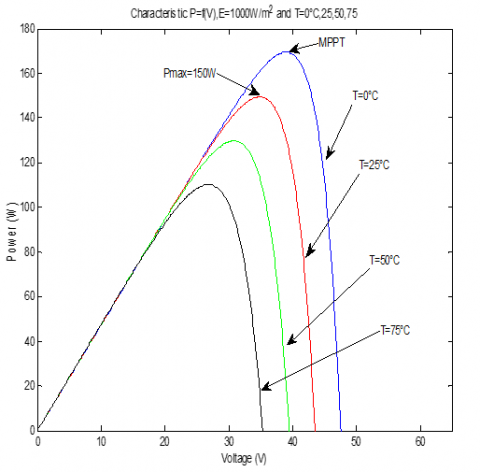Figure 3. Characteristic of PV, P=f(V)

## 4.png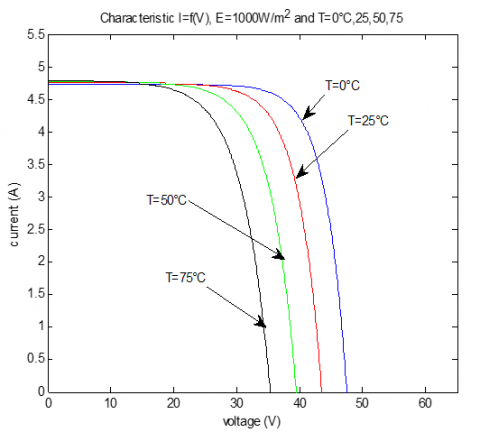Figure 4. Characteristic of PV, I=f(V)

5.1 Learning step

The following Figures 5 and 6 present the learning data and error obtaining of our ANFIS.

## 5.pngFigure 5. Training data

## 6.png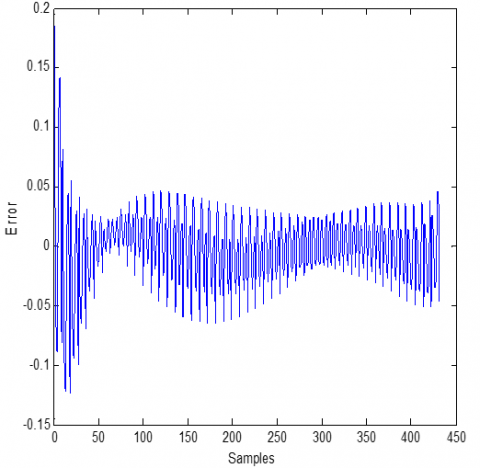Figure 6. Training error

The following Table 2 presents the architecture of our Model (ANFIS) for PV learning:

Table 2. ANFIS model architecture used to predict the MPPT

 Neuro-fuzzy model Training parameters Number of fuzzy rules 04 Training input $10^{\circ} \mathrm{C} \leq T \leq 80^{\circ} \mathrm{C}$ $100 \mathrm{~W} / \mathrm{m}^2 \leq G \leq 1000 \mathrm{~W} / \mathrm{m}^2$ Number of nodes 21 Number of linear parameters 12 Number of nonlinear parameters 12 Total number of parameters 24 Number of training data pairs 432 Mean Square Error (MSE) 0.0010

5.2 Validation step

Now we verify that our model after learning is actually able to predict the desired output for values given at the entry which are not used in the learning. We always should compare the true output of the networks with the model of the PV for comparisons using mean square error (MSE). For this we study three cases: two extreme cases and a general one.

5.2.1 Case 01: the irradiation varies and the temperature is constant as shown in Figures 7 and 8.

$\checkmark 100 \mathrm{~W} / \mathrm{m}^2 \leq E \leq 1000 \mathrm{~W} / \mathrm{m}^2$ and $T=2^{\circ} \mathrm{C}$

## 7.pngFigure 7.  '+' ANFIS model and 'O' PV panel MPPT for $T=2^{\circ} \mathrm{C}$ with MSE=0.17

$\checkmark \quad 50 \mathrm{~W} / \mathrm{m}^2 \leq E \leq 550 \mathrm{~W} / \mathrm{m}^2$ and $T=60^{\circ} \mathrm{C}$

## 8.png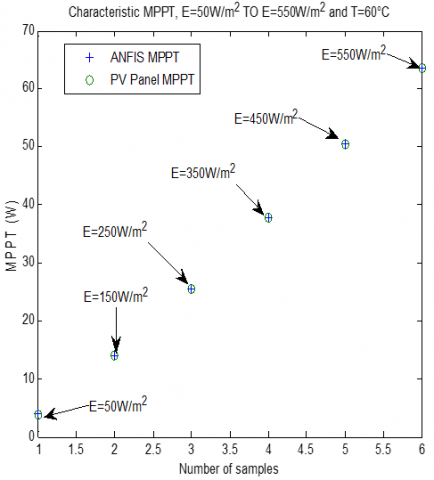Figure 8. ANFIS model and PV panel MPPT for $T=60^{\circ} \mathrm{C}$ with MSE=0.041

5.2.2 Case 02: The temperature varies and the irradiation is constant as shown in Figures 9 and 10.

$\checkmark \quad 25^{\circ} \mathrm{C} \leq T \leq 65^{\circ} \mathrm{C}$ and $E=900 \mathrm{~W} / \mathrm{m}^2$

## 9.pngFigure 9. ANFIS model and PV panel MPPT for $G=1000 \mathrm{~W} / \mathrm{m}^2$ with MSE= 7.5577e-004

$\checkmark \quad 30^{\circ} \mathrm{C} \leq T \leq 70^{\circ} \mathrm{C}$ and $E=700 \mathrm{~W} / \mathrm{m}^2$

## 10.png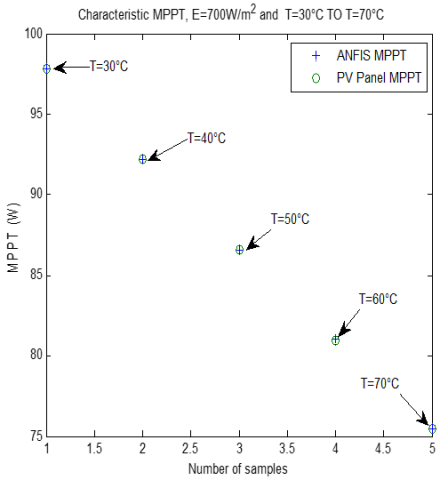Figure 10. ANFIS Model and PV Panel MPPT with MSE= 2.7117e-004

5.2.3 Case 03: The temperature varies and the irradiation varies too as presented in Figure 11.

$\checkmark \quad 0^{\circ} \mathrm{C} \leq T \leq 75^{\circ} \mathrm{C}$ and $100 \mathrm{~W} / \mathrm{m}^2 E \leq 850 \mathrm{~W} / \mathrm{m}^2$

## 11.png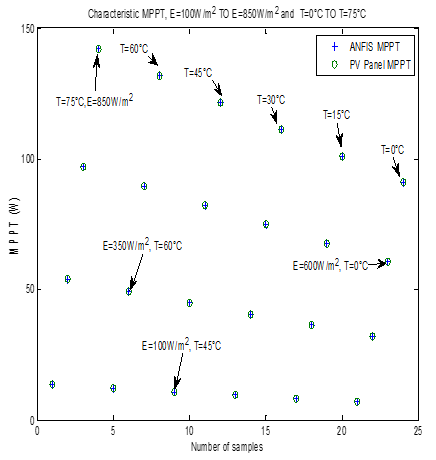Figure 11. '+' ANFIS model and 'O'+ PV Panel MPPT for T and E vary with MSE 1.6032e-004

By examining the errors of all the considered cases of the variation of the irradiation and the temperature, it can be seen that the implemented ANFIS is able to predict the MPPT of the PV panel for any set of values of the inputs (E,T) belonging to their prescribed domain of variations

6. Conclusion

The ANFIS model’s algorithm is presented to extract the maximum power from the PV Panel. The suggested approach is very efficient for creating sophisticated, nonlinear connections between a set input/output information.

Quickly and accurately ANFIS model can be produced from measured or simulated PV Panel data. Once generated, after having received the necessary training, for newly presented situations, a neuro-fuzzy system entirely eliminates using complex iterative processes again. In this paper. The simulation results demonstrated that the ANFIS technique performs well for following the maximum power point, its speed, and the reliability of its outputs in all studied cases.

The recommendations that can be made in the future investigation, is the real-time implementation of this algorithm and hardware testing are both possible in Python and C++. Moreover, design of a web source containing simulations of the PV system, the MPPT controller, and the most used and popular algorithms in MATLAB and other technologies. To test the recently created algorithms, it may be possible to develop and implement a reprogrammable MPPT controller in the hardware under an open license.  For this reason, we propose using 32-bit ARM microcontrollers.

References

 Reza, M., Hashim, H. Chandima, G. (2016). Power loss due to soiling on solar panel: A review. Renewable And Sustainable Energy Reviews. 59: 1307-1316. https://doi.org/10.1016/j.rser.2016.01.044

 Ibrahim, A. (2011). Effect of shadow and dust on the performance of silicon solar cell. J Basic Appl Sci Res, 1: 222-230.

 El-Nashar, A. (2009). Seasonal effect of dust deposition on a field of evacuated tube collectors on the performance of a solar desalination plant. Desalination, 239: 66-81. https://doi.org/10.1016/j.desal.2008.03.007

 Bendib, B., Belmili, H., Krim, F. (2015). A survey of the most used MPPT methods: Conventional and advanced algorithms applied for photovoltaic systems. Renew. Sustain. Energy Rev., 45: 637-648. https://doi.org/10.1016/j.rser.2015.02.009

 Kumar, D., Chatterjee, K. (2016). A review of conventional and advanced MPPT algorithms for wind energy systems. Renew. Sustain. Energy Rev., 55: 957-970. https://doi.org/10.1016/j.rser.2015.11.013

 Yu, K.N., Liao, C.K. (2015). Applying novel fractional order incremental conductance algorithm to design and study the maximum power tracking of small wind power systems. J. Appl. Res. Technol., 13: 238-244. https://doi.org/10.1016/j.jart.2015.06.002

 Borni, A., Abdelkrim, T., Bouarroudja, N., Bouchakoura, A., Zaghba, L., Lakhdari, A., Zarour., L. (2017). Optimized MPPT controllers using GA for grid connected photovoltaic systems, comparative study. Energy Procedia, 119: 278-296. Optimized MPPT Controllers Using GA for Grid Connected Photovoltaic Systems, Comparative study, Energy Procedia

 Abdelhalim, B., Bouarroudj, N., Bouchakour, A., Layachi, Z. (2017). P&O-PI and fuzzy-PI MPPT Controllers and their time domain optimization using PSO and GA for grid-connected photovoltaic system: A comparative study. Int. J. Power Electronics, 8(4): 300-322. http://dx.doi.org/10.1504/IJPELEC.2017.085199

 Jang, J.S.R. (1993). ANFIS: Adaptive-network-based fuzzy inference system. IEEE Trans Systems Man and Cybernetics, 23: 665. http://dx.doi.org/10.1109/21.256541

 Jang, J.S.R., Sun, C.T., Mizutani, E. (1997). Neuro-Fuzzy and Soft Computing: A Computational Approach to Learning and Machine Intelligence. Prentice-Hall, Upper Saddle River, NJ, 86(3). https://doi.org/10.1109/JPROC.1998.662886

 Al-ezzi, A.S. Nainar. M, Ansari, M. (2022). Photovoltaic solar cells: a review. applied system innovation. Appl. Syst. Innov., 5(4): 67. https://doi.org/10.3390/asi5040067

 Smith, R.P., Hwang, A.A.C., Beetz, T., Helgren, E. (2018). Introduction to semiconductor processing: Fabrication and characterization of p-n junction silicon solar cells. Am. J. Phys., 86: 740-746. http://dx.doi.org/10.1119/1.5046424

 Desoto, W., Klein, S., Beckman, W. (2006). Improvement and validation of a model for photovoltaic array performance. Solar Energy, 80: 78–88. https://doi.org/10.1016/j.solener.2005.06.010

 Masters, G.M. (2004). Renewable and Efficient Electric Power Systems. New Jersey: John Wiley& Sons. https://ieeexplore.ieee.org/book/5237268, accessed on Sept. 12, 2022.

 Kumar, C., Raj, T.D., Premkumar, M., Raj, T.D. (2020). A new stochastic slime mould optimization algorithm for the estimation of solar photovoltaic cell parameters. Optik, 223: 165277. https://doi.org/10.1016/j.ijleo.2020.165277

 Ndi, F.E., Perabi, S.N., Ndjakomo, S.E., Ondoua Abessolo, G., Mengounou Mengata, G. (2021). Estimation of single-diode and two diode solar cell parameters by equilibrium optimizer method. Energy Rep., 7: 4761-4768. https://doi.org/10.1016/j.egyr.2021.07.025

 Smets, A.H.M., Jager, K., Isabella, O., van Swaaij, R.A., Zeman, M. (2016). Solar cell parameters and equivalent circuit. Sol. Energy Phys. Eng. Photovolt. Convers. Technol. Syst, 113-121.

 Khezzar, R., Zereg, M., Khezzar, A. (2009). Comparative study of mathematical methods for parameters calculation of current-voltage characteristic of photovoltaic module. In International Conference on Electrical and Electronics Engineering, ELECO. https://doi.org/10.1109/ELECO.2009.5355236

 Abrar A˛ Asun C, Vadim, B. (2022). Modeling and Control Strategy of Wind Energy Conversion System with Grid-Connected Doubly-Fed Induction Generator. Energies, 15(18): 1-26. http://dx.doi.org/10.3390/en15186694

 Anudipta C, Rajkanya D, Muthuselvan P.K. (2022). Energy conversion strategies for wind energy system: Electrical, mechanical and material aspects. Materials, 15(3): 1-34. http://dx.doi.org/10.3390/ma15031232

 Kou, Q. Klein, S.A., Beckman, W.A. (1998). A method for estimating the long-term performance of direct-coupled PV pumping systems. Solar Energy, 64(1-3): 33-40. https://doi.org/10.1016/S0038-092X(98)00049-8

 Leedy, A.W. Garcia, K.E. (2014). An indirect method for maximum power point tracking for photovoltaic arrays. International Conference on Renewable Energy Research and Application (ICRERA): 19-22. https://doi.org/10.1109/ICRERA.2014.7016552

 Villalva, M.G., Gazoli, J.R., Ernesto, R.F. (2009). Analysis and simulation of the P&O MPPT algorithm using a linearized array model. Power electronics conference, Brazil: 189-195. https://doi.org/10.1109/IECON.2009.5414780

 Muhammad, K., Muhammad, M., Muhammad, R. (2020). Implementation of improved perturb & observe MPPT technique with confined search space for standalone photovoltaic system. Journal of King Saud University - Engineering Sciences, 32(7): 432-441. https://doi.org/10.1016/j.jksues.2018.04.006

 Azadeh, S., Mekhilef, S. (2011). Simulation and hardware implementation of incremental conductance MPPT with direct control method using cuk converter. IEEE Transaction on industrial electronics, 58(4): 1154-1161.

 Safari, A, Mekhilef, S. (2011). Implementation of incremental conductance method with direct control. IEEE region 10 conference ICON, Malaysia: 944-948. https://doi.org/10.1109/TENCON.2011.6129249

 Jawad, A. (2010). A fractional open circuit voltage based maximum power point tracker for photovoltaic arrays. 2nd international conference on software technology and engineering. IEEE. https://doi.org/10.1109/ICSTE.2010.5608868

 Nelson, D. Adriana, L. Duarte, O. (2011). Improved MPPT short-circuit current method by a fuzzy shor-circuit current estimator. IEEE: 211-218. https://doi.org/10.1109/ECCE.2011.6063771

 Adly, M., El-sherif, H., Ibrahim, M. (2011). Maximum power point tracker for a PV cell using fuzzy agent adapted by the fractional open Circuit Voltage Technique. IEEE International Conference on Fuzzy Systems, Taiwan:1918-1922. https://doi.org/10.1109/FUZZY.2011.6007697

 Nelson, D., Johann, H., Duarte, Or. (2010). Fuzzy MPP method improved by a short circuit current estimator, Applied to a grid- connected PV system. IEEE 12th Work Shop on Control and Modeling for Power Electronics: 1-6. https://doi.org/10.1109/COMPEL.2010.5562433

 Subiyanto, A., Mohamed, M., Hannan, A. (2009). Maximum power point tracking in grid connected PV system using a novel fuzzy logic controller. IEEE student conference on research and development: 349-352. https://doi.org/10.1109/SCORED.2009.5443002

 Mumtaz, S., Ahmad, S., Khan, L., Ali, S., Kamal, T., Hassan, S. (2018). Adaptive feedback linearization based neuro-fuzzy maximum power point tracking for a photovoltaic system. Energies, 11(3): 606. https://doi.org/10.3390/en11030606

 Bataineh, K., Eid, N. (2018). A hybrid maximum power point tracking method for photovoltaic systems for dynamic weather conditions. Resources, 7: 68. https://doi.org/10.3390/resources 7040068

 Zhang, C. Sun, J.G., Wang, Q.S., Feng, Z. (2007). A novel GA-LM based hybrid algorithm. Proc. ICNC: 479-483. https://doi.org/10.1109/ICNC.2007.112